Test: The Root Locus Technique- 2

# Test: The Root Locus Technique- 2 - Electrical Engineering (EE)

Test Description

## 10 Questions MCQ Test GATE Electrical Engineering (EE) 2024 Mock Test Series - Test: The Root Locus Technique- 2

Test: The Root Locus Technique- 2 for Electrical Engineering (EE) 2023 is part of GATE Electrical Engineering (EE) 2024 Mock Test Series preparation. The Test: The Root Locus Technique- 2 questions and answers have been prepared according to the Electrical Engineering (EE) exam syllabus.The Test: The Root Locus Technique- 2 MCQs are made for Electrical Engineering (EE) 2023 Exam. Find important definitions, questions, notes, meanings, examples, exercises, MCQs and online tests for Test: The Root Locus Technique- 2 below.
Solutions of Test: The Root Locus Technique- 2 questions in English are available as part of our GATE Electrical Engineering (EE) 2024 Mock Test Series for Electrical Engineering (EE) & Test: The Root Locus Technique- 2 solutions in Hindi for GATE Electrical Engineering (EE) 2024 Mock Test Series course. Download more important topics, notes, lectures and mock test series for Electrical Engineering (EE) Exam by signing up for free. Attempt Test: The Root Locus Technique- 2 | 10 questions in 30 minutes | Mock test for Electrical Engineering (EE) preparation | Free important questions MCQ to study GATE Electrical Engineering (EE) 2024 Mock Test Series for Electrical Engineering (EE) Exam | Download free PDF with solutions
 1 Crore+ students have signed up on EduRev. Have you?
Test: The Root Locus Technique- 2 - Question 1

### The open loop transfer function of a unity feedback control system is given by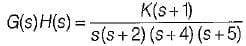The breakaway point in its root locus will lie between

Detailed Solution for Test: The Root Locus Technique- 2 - Question 1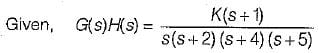Here, P = 4,Z = 1, P - Z = 3
No. of branches of RL terminating at zero = 1.
No. of branches of RL terminatina at infinity = 3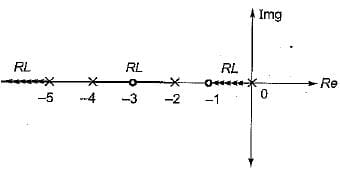Since out of the given option, real axis between s = -2 and s = -4 is only a part of root locus, therefore breakaway point will lie between -2 and -4.

Test: The Root Locus Technique- 2 - Question 2

### The characteristic equation of a closed loop control system is given by: s2 + 2s +10 + K(s2 + 6s + 10) = 0 The angle of asymptotes for the root loci for K ≥ 0 are given by

Detailed Solution for Test: The Root Locus Technique- 2 - Question 2

Given, s2 + 2s + 10 + K(s2 + 6s + 10) = 0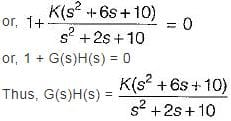Here, number of open loop poles, P = 2. Number of open loop zero, Z = 2
∴ P - Z = 0.
Angle of asymptotes are given by:Sines P - Z = 0, therefore there are no angle of asymptotes for the root locus of the given system.

Test: The Root Locus Technique- 2 - Question 3

### The breakaway point in the root loci plot for the loop transfer function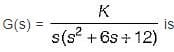Detailed Solution for Test: The Root Locus Technique- 2 - Question 3

Given,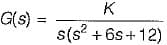1+ G(s) = 0
or, s (s2+ 6 s + 12) + K = 0
or, K = - (s3 + 6s2 + 12s)
For B.A. points,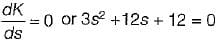or, s2 + 4s + 4 = 0
or, (s + 2)2 = 0
or, s = - 2 , - 2
For given O.L.T.F., P = 3, Z = 0, P - Z = 3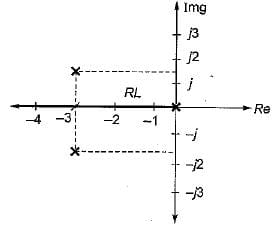Poles are at s = 0 and s = -3 ± j√3 = -3 ± j1.732
Since entire -ve real axis is a part of root locus, therefore s = -2 is a valid breakaway point.

Test: The Root Locus Technique- 2 - Question 4

Consider the following statements pertaining to root locus techniques:
1. Routh-Hurwitz criterion may be used to find the intersections of the root loci on the imaginary axis.
2. Breakaway points on the root loci of an equation corresponds to multiple-order roots of the equation.
3. The breakaway points on the root loci of 1 + KG(s) H(s) = 0 must satisfy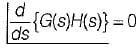4. n root loci arrive or depart a breakaway point at 180/n degrees.
Which of these statements are correct?

Test: The Root Locus Technique- 2 - Question 5

Assertion (A): From the root sensitivity standpoint, a system should not be operated at the breakaway points.
Reason (R): The root sensitivity at the breakaway points is zero.

Detailed Solution for Test: The Root Locus Technique- 2 - Question 5

From the root sensitivity, standpoint, a system should not be operated at the breakaway points because root sensitivity at breakaway point is infinite.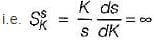at breakaway pointsHere, α = closed loop poles(s)
and β = system gain (K)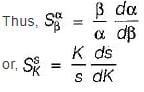Test: The Root Locus Technique- 2 - Question 6

Consider the following statements:
1. All the rules and properties for the construction of root loci in the s-plane can be applied to the construction of root loci of discrete data system in the z-plane without any modification.
2. The breakaway points of the root loci must always be on the real axis.
3. There is only, one intersection of the asymptotes of the complex root loci.
4. Adding a pole to Q(s)/P(s) has the general effect of pushing the root loci to the left, whereas adding a zero pushes the loci to the right.
Which of the above statements is/are not correct related to root locus technique in control system?

Detailed Solution for Test: The Root Locus Technique- 2 - Question 6

There is possibility of complex B.A. point. Also when a pole is added to OLTF, root locus pushes to right and reverse happens when a zero is added.

Test: The Root Locus Technique- 2 - Question 7

For the loop transfer function: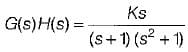the angle of departure at s = j for K > 0 is

Detailed Solution for Test: The Root Locus Technique- 2 - Question 7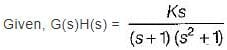Here, P = 3, Z = 1, P - Z = 2
No. of branches of RL terminating at zero = 1.
No. of branches of RL termination at infinity = 2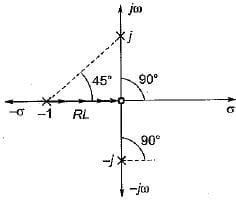Here, two branches of RL has to terminate at infinity.
Thus, the two imaginary poles will terminate at infinity.
Hence, we need to find angle of departure for which we join all poles and zeros with the imaainarv Dole at s = j1.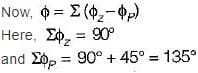Thus, φ = (90° -135°) = - 45°
∴  Angle of departure is,
φD = 180° + φ
= 180° - 45° = 135°

Test: The Root Locus Technique- 2 - Question 8

Which of the following is not true regarding the existence of breakaway point in root locus technique?

Test: The Root Locus Technique- 2 - Question 9

The open loop transfer function of a system is given by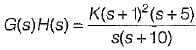The number of loci terminating at infinity is

Detailed Solution for Test: The Root Locus Technique- 2 - Question 9

Here,
P = 2, Z = 3 and Z > P
∴ No. of branches of RL terminating at infinity
= Z - P = 3 - 2 = 1

Test: The Root Locus Technique- 2 - Question 10

A unity feedback system has open-loop transfer function,The valid root-loci for this system is

Detailed Solution for Test: The Root Locus Technique- 2 - Question 10

To find whether root locus intersects the imaginary axis or not, we construct Routh’s array. Characteristic equation is
s3 + βs2 + Ks + Kα = 0
Routh's array is: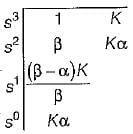For β > α > 0, root-loci doesn't intersect the jω-axis and is stable for all positive values of K (no sign change in first column for β > α > 0 and K> 0).
Also, P = 3, Z = 1, P - Z = 2
Thus, two branches of RL will terminate at infinity while one branch will terminate at a zero.
Therefore, possible root locus diagram will be represented by option (a).

## GATE Electrical Engineering (EE) 2024 Mock Test Series

23 docs|285 tests
Information about Test: The Root Locus Technique- 2 Page
In this test you can find the Exam questions for Test: The Root Locus Technique- 2 solved & explained in the simplest way possible. Besides giving Questions and answers for Test: The Root Locus Technique- 2, EduRev gives you an ample number of Online tests for practice

## GATE Electrical Engineering (EE) 2024 Mock Test Series

23 docs|285 tests